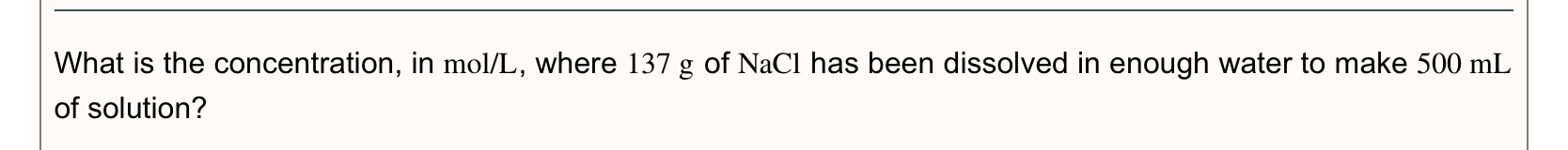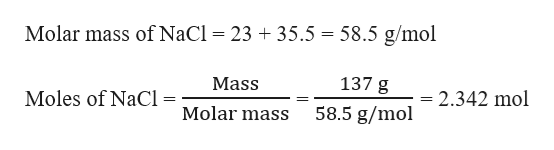# What is the concentration, in mol/L, where 137 g of NaCl has been dissolved in enough water to make 500 mLof solution?

Question
61 views

Questionhelp_outlineImage TranscriptioncloseWhat is the concentration, in mol/L, where 137 g of NaCl has been dissolved in enough water to make 500 mL of solution? fullscreen
check_circle

Step 1

Given,

Mass of NaCl = 137 g

Volume of solution = 500 mL = 0.5 L    (1 mL = 0.001 L)

Moles of NaCl can be calculated as:help_outlineImage TranscriptioncloseMolar mass of NaCl 23 35.5 58.5 g/mol = 137 g Mass Moles of NaCl = = 2.342 mol 58.5 g/mol Molar mass fullscreen
Step 2

The concentration in mol/L of the NaCl...

### Want to see the full answer?

See Solution

#### Want to see this answer and more?

Solutions are written by subject experts who are available 24/7. Questions are typically answered within 1 hour.*

See Solution
*Response times may vary by subject and question.
Tagged in

### Solutions